Processing ......FreeComputerBooks.com Links to Free Computer, Mathematics, Technical Books all over the World

Theory of Computation, Making Connections
🌠 Top Free Unix/Linux Books - 100% Free or Open Source!
• Title Theory of Computation, Making Connections
• Authors Jim Hefferon
• Publisher: Saint Michael's College; eBook (Creative Commons Licensed)
• Hardcover: N/A
• eBook: PDF (447 pages)
• Language: English
• ISBN-10: N/A
• ISBN-13: N/A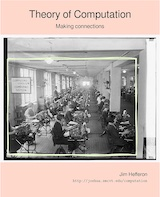Book Description

The book is geared toward those who thirst for computation theory knowledge. To cater to the demands of a wide range of people, the principles in this book are explained in a way that is easy to understand, digest and apply in the upcoming career.

The 'Theory of Computation' is the foundational and mathematical topic in computer science, computer applications, computer Engineering, and software engineering. This book provides a clear introduction to the fundamental principles, followed by an in-depth mathematical study and a wealth of solved problems. Before reading this book, learners must understand basic sets, functions, trees, graphs and strings.

The book as a whole acquaints the reader with automata theory fundamentals. The book provides theoretical coverage of the essential principles, solve instances, and solve multiple-choice problems with solutions. The theory and computation of automata presented in this book will greatly assist students and professors alike.

• Algorithmic ideas are made simple to understand through the use of examples.
• Contains a wide range of examples and solutions to help students better grasp the concepts.
• Designed to assist and coach students in applying the fundamentals of computation theory in real-world situations.
Reviews, Ratings, and Recommendations: Related Book Categories: Read and Download Links:Similar Books:
•Models of Computation: Exploring the Power of Computing

It covers the traditional topics of formal languages, automata and complexity classes, as well as an introduction to the more modern topics of space-time tradeoffs, memory hierarchies, parallel computation, the VLSI model, and circuit complexity.

•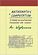Mathematics and Computation (Avi Wigderson)

This book provides a broad, conceptual overview of computational complexity theory - the mathematical study of efficient computation. With important practical applications to computer science and industry, computational complexity theory, etc.

•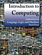Introduction to Computing: Language, Logic, and Machines

This book focuses on how to describe information processes by defining procedures, how to analyze the costs required to carry out a procedure, and the fundamental limits of what can and cannot be computed mechanically.

•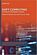Soft Computing: Techniques in Engineering Sciences

Soft Computing is used where a complex problem is not adequately specified for the use of conventional math and computer techniques. This book elaborates on the most recent applications of Soft Computing in various fields of engineering.

•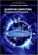Quantum Computing: Progress and Prospects

Provides an introduction to the Quantum Computing, including the unique characteristics and constraints of the technology, and assesses the feasibility and implications of creating a functional quantum computer capable of addressing real-world problems.

•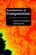Foundations of Computation (Carol Critchlow, et al)

It includes topics from discrete mathematics, automata theory, formal language theory, and the theory of computation, along with practical applications to computer science. The course has no prerequisites other than introductory computer programming.

•Introduction to Theory of Computation (Anil Maheshwari, et al)

This is a textbook on formal languages, automata theory, and computational complexity. This text strikes a good balance between rigor and an intuitive approach to computer theory.

•Computing Fundamentals: Theory and Practice of Software Design

The book introduces the reader to computer programming, i.e. algorithms and data structures. It covers many new programming concepts that have emerged in recent years including object-oriented programming and design patterns.

•Matters Computational: Ideas, Algorithms, Source Code

This book provides algorithms and ideas for computationalists, whether a working programmer or anyone interested in methods of computation. The implementations are done in C++ and the GP language, written for POSIX-compliant platforms.

•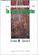Introduction to Theory of Computation (Eitan M. Gurari)

This book explores some of the more important terminologies and questions concerning programs, computers, problems, and computation. The exploration reduces in many cases to a study of mathematical theories, such as those of automata and formal languages; theories that are interesting also in their own right.

Book Categories
 :All CategoriesTop Free BooksRecent BooksMiscellaneous BooksComputer EngineeringComputer LanguagesComputer ScienceData Science/DatabasesJava and Java EE (J2EE)Linux and UnixMathematicsMicrosoft and .NETMobile ComputingNetworking and CommunicationsSoftware EngineeringSpecial TopicsWeb Programming
Other Categories